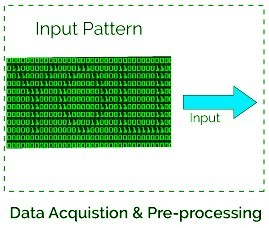Related Articles
CNN – Image data pre-processing with generators
• Last Updated : 16 Jul, 2020

The article aims to learn how to pre-processing the input image data to convert it into meaningful floating-point tensors for feeding into Convolutional Neural Networks. Just for the knowledge tensors are used to store data, they can be assumed as multidimensional arrays. A tensor representing a 64 X 64 image having 3 channels will have its dimensions (64, 64, 3). Currently, the data is stored on a drive as JPEG files, So let’s see the steps taken to achieve it.

Algorithm:

• Read the picture files (stored in data folder).
• Decode the JPEG content to RGB grids of pixels with channels.
• Convert these into floating-point tensors for input to neural nets.
• Rescale the pixel values (between 0 and 255) to the [0, 1] interval (as training neural networks with this range gets efficient).It may seem a bit fussy, but Keras has utilities to take over this whole algorithm and do the heavy lifting for you. Keras has a module with image-processing helping tools, located at `keras.preprocessing.image`. It contains the class ImageDataGenerator, which lets you quickly set up Python generators that can automatically turn image files on disk into batches of preprocessed tensors.

Code: Practical Implementation :

 `# Importing the ImageDataGenerator for pre-processing ``from` `keras.preprocessing.image ``import` `ImageDataGenerator`` ` `# Initialising the generators for train and test data``# The rescale parameter ensures the input range in [0, 1] ``train_datagen ``=` `ImageDataGenerator(rescale ``=` `1.``/``255``)``test_datagen ``=` `ImageDataGenerator(rescale ``=` `1.``/``255``)`` ` `# Creating the generators with each batch of size = 20 images``# The train_dir is the path to train folder which contains input classes``# Here it is 'cat' and 'dog' so class_mode is binary`` ` `train_generator ``=` `train_datagen.flow_from_directory(``                  ``train_dir,``                  ``target_size ``=``(``150``, ``150``),  ``# target_size = input image size``                  ``batch_size ``=` `20``,``                  ``class_mode ``=``'binary'``)`` ` ` ` `test_generator ``=` `test_datagen.flow_from_directory(``                    ``test_dir,``                    ``target_size ``=``(``150``, ``150``),``                    ``batch_size ``=` `20``,``                    ``class_mode ``=``'binary'``)`

Output:

```It yields batches of 150 × 150 RGB images of shape (20, 150, 150, 3)
and binary labels of shape (20, ).```

Fitting the model:
Let’s fit the model to the data using the generator, it is done using the fit_generator method, the equivalent of fit for data generators like given below. Its first argument is a Python generator that will yield batches of inputs and targets indefinitely because the data is being generated endlessly, the Keras model needs to know how many samples to draw from the generator before declaring an epoch over. This is the role of the steps_per_epoch argument.
Now deciding the steps_per_epoch parameter, as we have total of 2000 training images and each batch is of size 20, hence, the steps_per_epoch will be 2000 / 20 = 100.
Code:

 `# Your compiled model being trained with fit_generator``history ``=` `model.fit_generator(``             ``train_generator,``             ``steps_per_epoch ``=` `100``,``             ``epochs ``=` `30``,``             ``validation_data ``=` `test_generator,``             ``validation_steps ``=` `50``)`` ` `# Note: here the validation steps are necessary because``# the test_genrator also yield batches indefinitely in loops`My Personal Notes arrow_drop_up